Search by Topic

Resources tagged with Physics similar to Bridge Builder:

Filter by: Content type:
Age range:
Challenge level:

There are 78 results

Broad Topics > Applications > PhysicsLunar Leaper

Age 16 to 18 Challenge Level:

Gravity on the Moon is about 1/6th that on the Earth. A pole-vaulter 2 metres tall can clear a 5 metres pole on the Earth. How high a pole could he clear on the Moon?Cannon Balls

Age 16 to 18 Short Challenge Level:

How high will a ball taking a million seconds to fall travel?Ramping it Up

Age 16 to 18 Challenge Level:

Look at the calculus behind the simple act of a car going over a step.Neural Nets

Age 16 to 18

Find out some of the mathematics behind neural networks.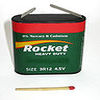Battery Modelling

Age 16 to 18 Challenge Level:

Find out how to model a battery mathematicallyMaths in the Undergraduate Physical Sciences

Age 16 to 18

An article about the kind of maths a first year undergraduate in physics, engineering and other physical sciences courses might encounter. The aim is to highlight the link between particular maths. . . .Pumping the Power

Age 16 to 18 Challenge Level:

What is an AC voltage? How much power does an AC power source supply?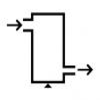Age 16 to 18 Challenge Level:

A look at a fluid mechanics technique called the Steady Flow Momentum Equation.Engnrich

Age 16 to 18 Challenge Level:

engNRICH is the area of the stemNRICH Advanced site devoted to the mathematics underlying the study of engineeringLevels of Bohr

Age 16 to 18 Challenge Level:

Look at the units in the expression for the energy levels of the electrons in a hydrogen atom according to the Bohr model.The Lorentz Force Law

Age 16 to 18 Challenge Level:

Explore the Lorentz force law for charges moving in different ways.Striking Gold

Age 16 to 18 Challenge Level:

Investigate some of the issues raised by Geiger and Marsden's famous scattering experiment in which they fired alpha particles at a sheet of gold.The Amazing Properties of Water

Age 14 to 18 Challenge Level:

Find out why water is one of the most amazing compounds in the universe and why it is essential for life. - UNDER DEVELOPMENTBig and Small Numbers in Chemistry

Age 14 to 16 Challenge Level:

Get some practice using big and small numbers in chemistry.Stemnrich - Technology

Age 11 to 16 Challenge Level:

This is the technology section of stemNRICH - Core.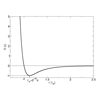Lennard Jones Potential

Age 16 to 18 Challenge Level:

Investigate why the Lennard-Jones potential gives a good approximate explanation for the behaviour of atoms at close ranges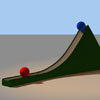Whoosh

Age 16 to 18 Challenge Level:

A ball whooshes down a slide and hits another ball which flies off the slide horizontally as a projectile. How far does it go?Gravity Paths

Age 16 to 18 Challenge Level:

Where will the spaceman go when he falls through these strange planetary systems?Ideal Gases

Age 16 to 18 Challenge Level:

Problems which make you think about the kinetic ideas underlying the ideal gas laws.The Not-so-simple Pendulum 1

Age 16 to 18 Challenge Level:

See how the motion of the simple pendulum is not-so-simple after all.Escape from Planet Earth

Age 16 to 18 Challenge Level:

How fast would you have to throw a ball upwards so that it would never land?Motorbike Momentum

Age 16 to 18 Challenge Level:

A think about the physics of a motorbike riding upside downMach Attack

Age 16 to 18 Challenge Level:

Have you got the Mach knack? Discover the mathematics behind exceeding the sound barrier.Core Scientific Mathematics

Age 14 to 18 Challenge Level:

This is the area of the advanced stemNRICH site devoted to the core applied mathematics underlying the sciences.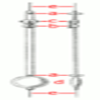The Not-so-simple Pendulum 2

Age 16 to 18 Challenge Level:

Things are roughened up and friction is now added to the approximate simple pendulumConstantly Changing

Age 14 to 16 Challenge Level:

Many physical constants are only known to a certain accuracy. Explore the numerical error bounds in the mass of water and its constituents.New Units for Old

Age 16 to 18 Challenge Level:

Can you match up the entries from this table of units?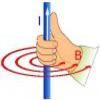Electromagnetism

Age 16 to 18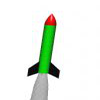Go Spaceship Go

Age 16 to 18 Challenge Level:

Show that even a very powerful spaceship would eventually run out of overtaking powerApproximately Certain

Age 14 to 18 Challenge Level:

Estimate these curious quantities sufficiently accurately that you can rank them in order of sizeMoving Stonehenge

Age 16 to 18 Challenge Level:

A look at the fluid mechanics questions that are raised by the Stonehenge 'bluestones'.Drug Stabiliser

Age 16 to 18 Challenge Level:

How does the half-life of a drug affect the build up of medication in the body over time?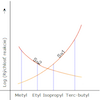Reaction Types

Age 16 to 18 Challenge Level:

Explore the rates of growth of the sorts of simple polynomials often used in mathematical modelling.Powerfully Fast

Age 16 to 18 Challenge Level:

Explore the power of aeroplanes, spaceships and horses.The Ultra Particle

Age 16 to 18 Challenge Level:

Explore the energy of this incredibly energetic particle which struck Earth on October 15th 1991Pack Man

Age 16 to 18 Challenge Level:

A look at different crystal lattice structures, and how they relate to structural propertiesThe Power of Dimensional Analysis

Age 14 to 18

An introduction to a useful tool to check the validity of an equation.Modelling Assumptions in Mechanics

Age 16 to 18

An article demonstrating mathematically how various physical modelling assumptions affect the solution to the seemingly simple problem of the projectile.Big and Small Numbers in Physics

Age 14 to 16 Challenge Level:

Work out the numerical values for these physical quantities.Ideal Axes

Age 16 to 18 Challenge Level:

Explore how can changing the axes for a plot of an equation can lead to different shaped graphs emergingEudiometry

Age 16 to 18 Challenge Level:

When a mixture of gases burn, will the volume change?Diamonds Aren't Forever

Age 16 to 18 Challenge Level:

Ever wondered what it would be like to vaporise a diamond? Find out inside...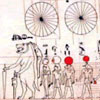Ancient Astronomical Terms

Age 11 to 18

Some explanations of basic terms and some phenomena discovered by ancient astronomersSweeping Satellite

Age 16 to 18 Challenge Level:

Derive an equation which describes satellite dynamics.Universal Time, Mass, Length

Age 16 to 18 Short Challenge Level:

Can you work out the natural time scale for the universe?Cobalt Decay

Age 16 to 18 Challenge Level:

Investigate the effects of the half-lifes of the isotopes of cobalt on the mass of a mystery lump of the element.Big and Small Numbers in Physics - Group Task

Age 16 to 18 Challenge Level:

Work in groups to try to create the best approximations to these physical quantities.Earth Orbit

Age 16 to 18 Challenge Level:

Follow in the steps of Newton and find the path that the earth follows around the sun.Resistance

Age 16 to 18 Challenge Level:

Find the equation from which to calculate the resistance of an infinite network of resistances.Motion Capture

Age 11 to 16 Challenge Level:

Explore displacement/time and velocity/time graphs with this mouse motion sensor.